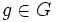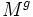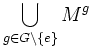# Effective action of finite group of diffeomorphisms has free point

(diff) ← Older revision | Latest revision (diff) | Newer revision → (diff)
Jump to: navigation, search

## Statement

Suppose$M$ is a connected differential manifold and$G$ is a finite subgroup of the self-diffeomorphism group of$M$. In other words,$G$ is a finite group acting on$M$ such that no non-identity element of$G$ acts trivially on$M$.

Then, the action of$G$ on$M$ has a free point: a point whose isotropy subgroup (stabilizer) is trivial.

## Definitions used

Fill this in later

## Facts used

We use two main facts:

1. Fixed-point set of finite group of diffeomorphisms is closed submanifold
2. A finite union of submanifolds of codimension at least one is proper.

## Proof

For every$g \in G$ other than the identity element, consider the set$M^g$ of elements of$M$ fixed by the cyclic subgroup generated by$g$. By fact (1) above, this is a closed submanifold, and by the effectiveness assumption, it cannot be the whole of$M$. Since$M$ is connected, it cannot be of full dimension, otherwise it would be both closed and open. Hence$M^g$ is either empty, or is a submanifold of codimension at least$1$.

Now, consider:$\bigcup_{g \in G \setminus \{ e \}} M^g$

This is a finite union of submanifolds of codimension at least 1, so is a proper subset. Thus, we can pick a point not fixed by any$g \in G$, and this is the desired free point.